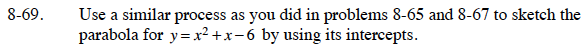### Home > CCA > Chapter Ch8 > Lesson 8.2.2 > Problem8-69

8-69.

Use a similar process as you did in problems 8-65 and 8-67 to sketch the parabola for y = x2 + x − 6 by using its intercepts. Homework Help ✎The y-intercept occurs when x = 0 and the x-intercepts occur when y = 0.

y-intercept
y = 02 + 0 − 6

x-intercepts
x2 + x − 6 = 0

There are two x-intercepts and one y-intercept.
Remember to sketch the graph.